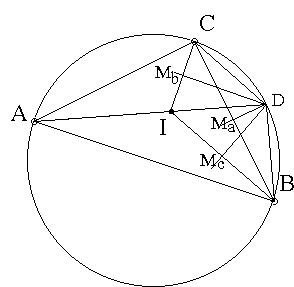# A problem with incircles and circumcircles What is it about? A Mathematical Droodle

Created with GeoGebra

Explanation### Explanation

Let I be the incenter of ΔABC. Consider 3 triangles: IBC, ICA, and IAB. An interesting fact is that the circumcenters of these triangles lie on the circumcircle of ΔABC.Indeed, let D be the circumcenter of ΔIBC. D lies at the intersection of the perpendicular bisectors MaD, MbD, and McD of sides BC, IC, and IB. We want to show that D lies on the circumcircle of ΔABC.

Angles MaDMc and IBC have pairwise perpendicular sides. Therefore they are equal. And similarly for angles MaDMb and ICB:

From (1) we obtain

 ∠MaDMc + ∠MaDMb = ∠MbDMc = ∠IDMb + ∠IDMc = ∠IDC/2 + ∠IDB/2 = ∠CDB/2

Comparing this to (1) gives ∠CDB = ∠C + ∠B. In other words, ∠CDB + ∠A = 180°, which exactly means that D lies on the circumcircle of ΔABC.

It is also clear that the circumcenter D of ΔBIC lies on bisector AI of ∠BAC.

### Remark

A weaker variant of the problem has been offered at the 1988 USA Olympiad where it was required to prove that the circumcircles of ΔABC and ΔOAOBOC are concentric.

In [Johnson, p. 185-185, 292°] the problem appears thus: Let D be the intersection of the A-bisector of ΔABC with the circumcircle. Then the circle centered at D with the radius equal to DB (= DC) passes through the incenter I.

### References

1. R. Honsberger, From Erdös To Kiev, MAA, 1996, pp. 56-57.
2. R. A. Johnson, Advanced Euclidean Geometry (Modern Geometry), Dover, 1960